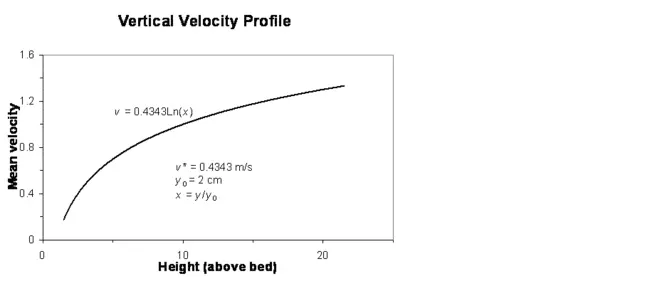Vertical Velocity Profile in Turbulent FlowView Original Image at Full Size

The mean stream velocity as a function of height above the bed. The surface roughness is normally the height at which the velocity becomes zero, but the height here is given in multiples of surface roughness, i.e., x = 10 implies y = 20.

Image 18801 is a 395 by 913 pixel WebP